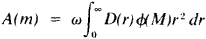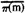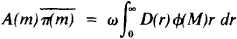# stellar statistics

## stellar statistics

The use of statistical methods in the study of spatial and temporal distributions and motions of celestial objects within a galaxy, region of the Universe, etc., and of spatial and temporal distributions of certain features or of certain categories of these objects. The number of stars in a galaxy can be of the order of 1011 and the number of galaxies runs into countless millions. Only a very small number of stars, galaxies, radio sources, etc., can be examined in any detail and from this sample, analyzed statistically, a more general picture emerges. Much of the data that is used comes from star catalogs, source counts, or detailed studies of selected areas. Almost all the information concerning the shape and structure of our Galaxy and the spatial distribution of other galaxies and of clusters of galaxies has been determined statistically.
Collins Dictionary of Astronomy © Market House Books Ltd, 2006
The following article is from The Great Soviet Encyclopedia (1979). It might be outdated or ideologically biased.

## Stellar Statistics

a branch of stellar astronomy that, using the methods of mathematical statistics, studies the space distribution of stars with similar physical characteristics and various statistical relationships among these stellar characteristics. The foundation of stellar statistics was laid by W. Herschel, who at the end of the 18th century, discovered an increase in the number of stars visible in his telescope as one approached the plane of the Milky Way (the galactic concentration) and explained this by the flattening of our galaxy. One of the important tasks of stellar statistics is the determination of the star density D(r) —that is, the number of stars per unit volume in a given direction at a distance r. Statistical methods are mostly used for the solution of this problem, since it is possible to directly determine the distance either to objects nearest the sun (r < 100 parsec) or to certain special types of stars—for example, variable stars.

Extensive use has been made in stellar statistics of the differential distribution function of stars according to their apparent stellar magnitude A(m) and the integral function N(m) showing the number of stars brighter than a given magnitude m , as well as of the distribution function of stars according to their absolute magnitude, the luminosity function φ(M). The functions A(m) and N(m) are directly determined by counts of stars of a given apparent magnitude or of stars brighter than this magnitude. It is possible to determine the luminosity function by solving the integral equations of stellar statistics.

The function A(m) is related to the star density function D(r) and the lumnosity function φ(M) by the following formula (the first integral equation of stellar statistics):where ω is a selected solid angle. With the help of the mean parallaxof stars with apparent magnitude m, the following relation is derived (the second integral equation of stellar statistics):These equations are used to determine both D(r) and ω(M). The equations of stellar statistics are mostly solved by numerical methods. The two equations mentioned are termed the Schwarzschild equations, named for the German astronomer K. Schwarzschild, who derived them in 1910.

Assuming the existence of interstellar absorption of light, the integral equations preserve their form; however, as a result of their solution one obtains an apparent star density D ‘(r) with the help of which, if the dependence of Ight absorption upon distance is known, that is, the light absorption function A(r), it is possible to determine the true star density D(r)

A convenient method in studying the distribution of celestial objects is the one proposed in 1937 by the Soviet astronomer M. A. Vashakidze and, independently, by the Dutch astronomer J. Oort in 1938. This method makes it possible to study the distribution of the star density in an arbitrary direction if its distribution is known in the direction perpendicular to the galactic plane. Thus it has been established that the star density has a general tendency to increase in the direction toward the center of our galaxy, and the sun is situated between two local concentrations, which can be identified with the spiral arms of our galaxy.

The Vashakidze-Oort method was used by the Soviet astronomer B. V. Kukarkin in 1947 to investigate the space distribution of variable stars. It was shown that different types of variable stars are characterized by different degrees of concentration toward the plane of the Milky Way Galaxy and toward the galactic center, and the parameters of the space distribution of the stars are connected with their kinematic characteristics.

E. D. PAVLOVSKAIA